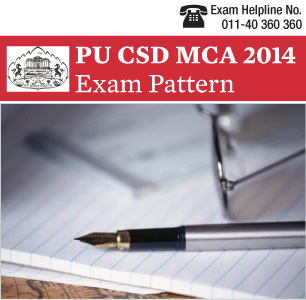PUCSD MCA Entrance Exam Pattern 2014:  Pune University Computer Science Department will be conducting the university level computer application entrance examination PU CSD MCA on March 23, 2014. Qualifying candidates will secure admission to full time MCA courses for the current academic year 2014.

For queries and doubts, call PU CSD MCA 2014 Exam Helpline at 011-40360360

Detail information regarding total number of questions, marking scheme and important topics of PU CSD MCA Entrance Exam 2014 Paper Pattern is given in this article so as to enhance exam preparation of candidates. Applicants need to check here for PU CSD MCA Entrance Exam 2014 Paper Pattern, which is given below.

The question paper of PU CSD MCA Entrance Exam 2014 will contain 100 multiple choice questions (MCQs). Each question will carry one mark.

For PU CSD MCA 2014 Entrance Exam following subjects -Mathematics, General Aptitude and English language will be covered.

Mathematics Syllabus and a few important topics of the subject are presented below in a box.

 Chapters Important Topics Set theory Set operations, relations, functions. Propositional logic Formulation, deduction, evaluation, puzzles Linear Algebra Solution of a system of linear equations. Determinant and inverse of a matrix, basic properties of matrices. Co-ordinate Geometry & Conic Sections Equations of lines and planes, vector products; definitions and properties of conic sections. Trigonometry Identities, computation of heights and lengths. Differential Calculus Total and partial differentiation, limit of a function. Integral Calculus Definite and indefinite integrals, solution of differential equations, computation of areas and volumes. Linear Algebra Solution of a system of linear equations. Determinant and inverse of a matrix, basic properties of matrices. Series and sequences Sum, mean, convergence, limit. Real and complex numbers Surds, solution of equations on complex domain. Polynomials Solution of quadratic equations, properties of roots of polynomials with real coefficients, binomial expansion, Taylor series. Elementary Probability Theory Computing probability from combinatorial reasoning, conditional probability.

Stay tuned to it.careers360.com for more news and articles on PU CSD MCA 2014 Exam Pattern

Note: Admissions of qualifying candidates for MCA course for the academic session 2014 will be done in accordance with the Ordinance No. 181 of section 53 (i) and section 65 of Maharashtra Universities Act 1994.

Questions related to Pune University Entrance Exam

Showing 1 out of 1 Questions
26 Views

pune University entrance exam 2020

Vundela Vidya Sree Student Expert 11th Oct, 2019Hello aspirant!!
To get admission into Pune University then you have appear for Entrance exam of Pune University. You should get good rank to get admission into this University. So prepare well for Pune University entrance exam 2020 to get seat inthis University in the branch you want to study Engineering.
Top
The question have been saved in answer later, you can access it from your profile anytime. Access now